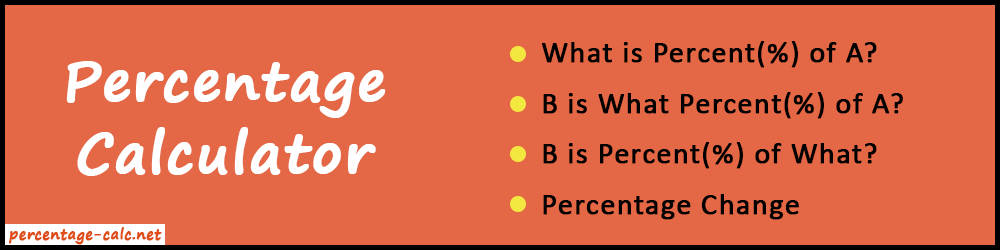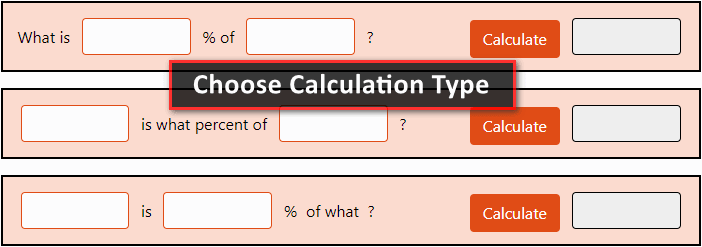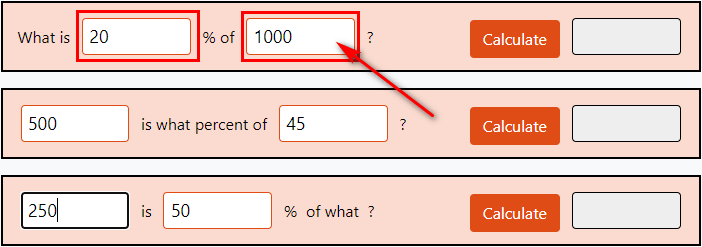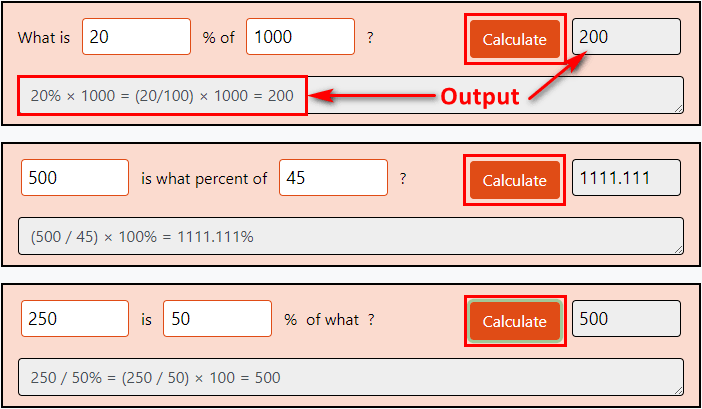# Percentage Calculator Online

Percentage calculator is a free online tool that allows you to find the percentage change, difference, and increase or decrease online.

##### Percent to Fraction or Decimal Conversion:
 Percent: Fraction: Decimal: %Do you know the most interesting thing about this Percentage Calculator? We have used 4 most common phrases here for better understanding. Also, you can use any part of the tool free of cost without any problem. As you can see, the user interface has a lot of things. But don't worry about it. The user experience remains simple and smooth. That's why we have included phrases to tell about the functionality. So, users can easily find the percent(%) values as per their need.

In addition, you don't need to think or remember the formulas to do the calculation. Just enter the values inside the calculator and get the results very fast. Also, in this article, you will get important information regarding the percentage calculation with formulas and examples. Just stay tuned and read the full article.

### What is Percentage?

The word "Percentage" has been derived from the Latin word "Per Centum" that means "By a Hundred". When we say percent, we actually say "Per Hundred".

Defination: It is a number or ratio that defines a fraction of 100. Its symbol is "%" or "Percent".

Generally, we use these following terms so many times in our routine life.

• This water bottle is 60% empty.
• Next month, I will get a 25% increment on my salary.
• Tom got 95% in physics class.

In other words, it is the best way to represent "How much" or "How many". Even more, you can use it to show changes, increase or decrease ratio, difference, and a portion of any physical thing.

Also, in statistics, the percentage is very important. Because it sounds more technical to say 50% rather than saying 0.5. Therefore, it is a more convenient way to represent fractions.

## How do I Calculate a Percentage?

There are many formulas and solutions to find the percentage manually. But the most common formula is as below.

##### Percentage Formula:
 A B
=   P × 100

Where,

• P = Percentage
• A = First value
• B = Second Value

From this formula, we can make many variations. But we have only used four most common variations in our percentage calculator. Because they are used in the majority of cases.

The variations are:

Now, let's discuss its formula with some examples one by one.

Also, don't worry about remembering the formulas and calculations. Because it is for learning and knowledge purpose. In regular usage, you can use our percentage calculator and get rid of these manual calculations.

#### 1. What is Percent(%) of A?

Formula: P% × A = B

Let's take an example for more clarification.

##### Example: What is 18% of 120?

Firstly, let's differentiate the values from the question.

In our case,

• P = 18%
• A = 120
• B = ? (Find Out)

Place above all values in the formula:

18% × 120 = B

Now firstly, we have to convert percentage value into decimal. For that, we can remove the % sign and divide the percentage value with 100.

 18 100
=   0.18

Place the decimal value in the equation and multiply with A value.

0.18 × 120 = 21.6

Finally we get, B = 21.6

#### 2. B is what percent(%) of A?

Formula:
 B A
=   P%
##### Example: 25 is what percent of 150?

Here,

• A = 150
• B = 25
• P(%) = ?

So, the equation will be:

 25 150
=   0.167

Note: The above result is in decimal form. So, we need to multiply the result by 100 to convert it into a percent.

0.167 × 100 = 16.7%

Finally we get, P = 16.7%

#### 3. B is percent(%) of what?

Formula:
 B P%
=   A
##### Example: 30 is 15% of what?

In this case,

• B = 30
• P = 15%
• A = ?

Let's place above all values in the formula:

 30 15%
=   A

Firstly, convert the 15% into decimal. So, we will divide the 15 by 100.

 15 100
=   0.15

Now get back to the formula and place the decimal value.

 30 0.15
=   200

Therefore, A = 200.

#### 4. Percentage Change

Using this formula, we can find the percent change between the two values.

Formula:
 B - A A
=   P%
##### Example: What is percentage change from 35 to 55?

Here,

• A = 35
• B = 55
• P = ?
 55 - 35 35
=
 20 35
=   0.571

Now convert the 0.571 into percent.

0.571 × 100 = 57.1%

So, P = 57.1%

#### 5. Percent, Decimal, and Fraction Conversion

You can convert any percentage values into fractions or decimals using our tool. This feature is at the bottom of the calculator. Even more, it allows you to do the reverse conversion too. For example, fraction to decimal to percent or decimal to fraction to percent. So, we can find any values in between these three.

50%   =
 1 2
=   0.5

#### Features of Percentage Calculator

• Simple to use UI: The tool is not very complex to use. Everything is crystal clear. Just put the values and get the answers.
• Fast Results: The calculator gives you a resultant output very fast. No page loading issue. Also, you can run our calculator with a slow internet connection.
• Effortless: No need to do the boring manual mathematical calculations. It will save you time and effort.
• 100% Accurate: Our tool will never give you an incorrect answer. Because it runs on a pre-defined set of algorithms. Also, we have tested it in all possible ways. So, there is no chance for mistakes or wrong output.

### How to use Percentage Calculator?

Nowadays, online users always need efficient, all-time handy, accurate, and easy-to-use calculators. So, they can use it anytime and anywhere. Our tool stands out to be the best in all these terms. Also, every part of the calculator has its own functionality and everything is free to use. Let's know how to use them.

We have developed 5 different sections in Percentage Calculator. Each has different mechanisms. But the usage process is similar for all. So, here we will discuss only one section. Later you can follow the same process to operate others.

##### 1. Choose Calculation Type

When you open the website, you can see many blank input boxes. So, firstly, you have to decide what type of calculation do you want to do. There are many phrases available. So, choose anyone.##### 2. Input the Values

Then you can input the values or numbers inside the empty boxes.##### 3. Calculate and Reset

Lastly, press the "Calculate" button. As a result, you will get the conversion result below with full math calculation. Also, you can empty all the fields using the "Reset All" button. It will refresh the calculator.Repeat the same process for other phrases. That's it.

### Conclusion

Above all information was about the Percentage Calculator. Now, you know the answer to "How do I Calculate a Percentage?". This web application has all the things that you need from a basic to an advanced level. No charges or hidden cost to using it. Also, there is no usage limit. So, use this as many times as you want.